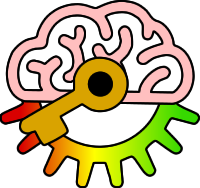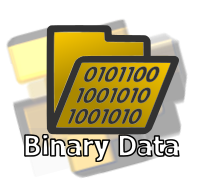# Y8

4m: Recall that binary digits represent all information that is stored, transmitted, and processed by computers.

Keywords:Test your self on these keywords and definitions using the games below or
KeywordDefinition
ASCIIAmerican Standard Code for Information Interchange (a way of converting each letter of the latin alphabet into a number so it can be represented in binary)
bitmap imagecan be represented by binary digits by describing the colour of each using numbers and then converting each number into binary
numberscan be represented in binary by converting from decimal to binary
soundcan be represented by binary digits by regularly sampling the amplitude (size) of a sound wave and converting each sample into binary
textcan be represented by binary digits by giving each letter a numerical value then converting that into binary
vector imagecan be represented by binary digits by describing each shape and colour using numbers and then converting each number into binary
videocan be represented by binary digits by splitting it into frames of still images and describing each frame as a sequence of coloured pixels and sound
Keyword games:
Resources: